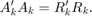# Implement Hardware-Efficient Real Partial-Systolic Q-less QR with Forgetting Factor

This example shows how to use the hardware-efficient Real Partial-Systolic Q-less QR Decomposition with Forgetting Factor block.

### Q-less QR Decomposition with Forgetting Factor

The Real Partial-Systolic Q-less QR Decomposition with Forgetting Factor block implements the following recursion to compute the upper-triangular factor R of continuously streaming n-by-1 row vectors A(k,:) using forgetting factor. It's as if matrix A is infinitely tall. The forgetting factor in the rangekeeps it from integrating without bound.### Define System Parameters

n is the length of the row vectors A(k,:) and the number of rows and columns in R.

n = 5; 

m is the effective numbers of rows of A to integrate over.

m = 100; 

Use the fixed.forgettingFactor function to compute the forgetting factor as a function of the number of rows that you are integrating over.

forgettingFactor = fixed.forgettingFactor(m) 
forgettingFactor = 0.9950 

precisionBits defines the number of bits of precision required for the QR Decomposition. Set this value according to system requirements.

precisionBits = 24; 

In this example, real-valued matrix A is constructed such that the magnitude of its elements are less than or equal to one. Your own system requirements will define what those values are. If you don't know what they are, and A is a fixed-point input to the system, then you can use the upperbound function to determine the upper bounds of the fixed-point types of A.

max_abs_A is an upper bound on the maximum magnitude element of A.

max_abs_A = 1; 

### Select Fixed-Point Types

Use the fixed.qlessqrFixedpointTypes function to compute fixed-point types.

T = fixed.qlessqrFixedpointTypes(m,max_abs_A,precisionBits) 
T = struct with fields: A: [0x0 embedded.fi] 

T.A is the fixed-point type computed for transforming A to R in-place so that it does not overflow.

T.A 
ans = [] DataTypeMode: Fixed-point: binary point scaling Signedness: Signed WordLength: 31 FractionLength: 24 

### Define Simulation Parameters

Create random matrix A to contain a specified number of inputs.

numInputs is the number of input rows A(k,:) for this example.

numInputs = 500; rng('default') A = fixed.example.realUniformRandomArray(-1,1,numInputs,n); 

Cast the inputs to the types determined by fixed.qlessqrFixedpointTypes.

A = cast(A,'like',T.A); 

Cast the forgetting factor to a fixed-point type with the same word length as A and best-precision scaling.

forgettingFactor = fi(forgettingFactor,1,T.A.WordLength); 

Select a stop time for the simulation that is long enough to process all the inputs from A.

stopTime = 2*numInputs*T.A.WordLength; 

### Open the Model

model = 'RealPartialSystolicQlessQRForgettingFactorModel'; open_system(model);### Set Variables in the Model Workspace

Use the helper function setModelWorkspace to add the variables defined above to the model workspace.

fixed.example.setModelWorkspace(model,'A',A,'n',n,... 'forgettingFactor',forgettingFactor,... 'regularizationParameter',0,... 'stopTime',stopTime); 

### Simulate the Model

out = sim(model); 

### Verify the Accuracy of the Output

Define matrixas followsThen using the formula for the computation of theth output, and the fact that, you can show thatSo to verify the output, the difference betweenandshould be small.

Choose the last output of the simulation.

R = double(out.R(:,:,end)) 
R = 5.2822 0.0429 -0.1900 0.4328 -0.0822 0 5.5459 0.0530 0.0010 -0.6132 0 0 5.4749 0.0189 -0.1018 0 0 0 5.6960 -0.1255 0 0 0 0 5.5661 

Verify that R is upper triangular.

isequal(R,triu(R)) 
ans = logical 1 

Verify that the diagonal is greater than or equal to zero.

diag(R) 
ans = 5.2822 5.5459 5.4749 5.6960 5.5661 

Synchronize the last output R with the input by finding the number of inputs that produced it.

A = double(A); alpha = double(forgettingFactor); relative_errors = nan(1,n); for k = 1:numInputs A_k = alpha.^(k:-1:1)' .* A(1:k,:); relative_errors(k) = norm(A_k'*A_k - R'*R)/norm(A_k'*A_k); end 

k is the number of inputs A(k,:) that produced the last R.

k = find(relative_errors==min(relative_errors),1,'last') 
k = 497 

Verify thatwith a small relative error.

A_k = alpha.^(k:-1:1)' .* A(1:k,:); relative_error = norm(A_k'*A_k - R'*R)/norm(A_k'*A_k) 
relative_error = 8.0991e-06 

Suppress mlint warnings in this file.

%#ok<*NOPTS>